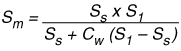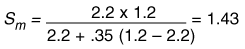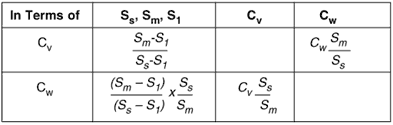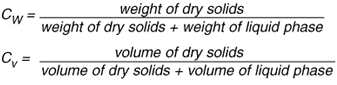### UsefulFormulasSection1

Useful Formulas Section 1

a. The formula for specific gravity of a solids-liquids mixture or slurry, Sm is:where,

S m = specific gravity of mixture or slurry
S i = specific gravity of liquid phase
S s = specific gravity of solids phase
C w = concentration of solids by weight
C v = concentration of solids by volume
EXAMPLE: if the liquid has a specific gravity of 1.2 and the concentration of solids by weight is 35% with the solids having a specific gravity of 2.2, then:b. Basic relationships among concentration and specific gravities of solid liquid mixtures are shown below:Where pumps are to be applied to mixtures which are both corrosive and abrasive, the predominant factor causing wear should be identified and the materials of construction selected accordingly. This often results in a compromise and in many cases can only be decided as a result of test or operational experience.

For any slurry pump application a complete description of the mixture components is required in order to select the correct type of pump and materials of construction.c. Slurry flow requirements can be determined from the expression:EXAMPLE: 2,400 tons of dry solids is processed in 24 hours in water with a specific gravity of 1.0 and the concentration of solids by weight is 30% with the solids having a specific gravity of 2.7 then: